# Dependent Variable – Examples, PDF

Every good research statement will need a well-grounded independent variable and a researched dependent variable. The dependent variable provides the main idea, context, theme, and tone of the research. This is one of the pivotal elements of the scientific method.

## 1. Type of Dependent Variable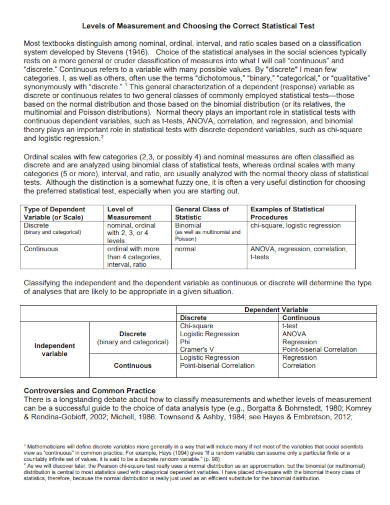web.pdx.edu

## 2. Continuous Dependent Variable Models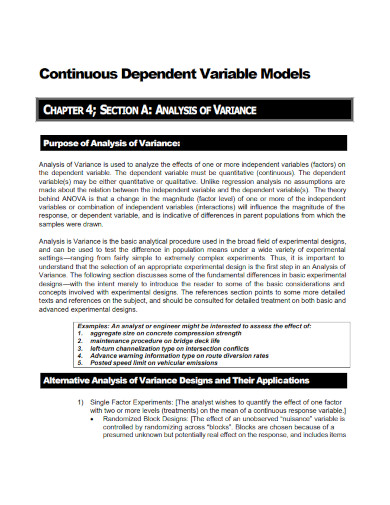onlinepubs.trb.org

## 3. Models for Binary Dependent Variable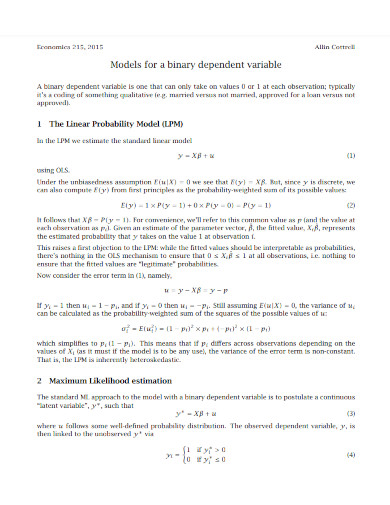users.wfu.edu

## 4. Sampling on Dependent Variable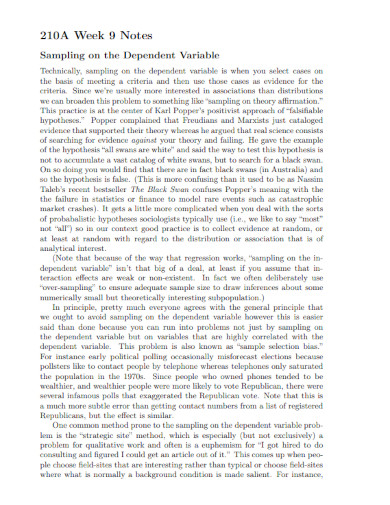gabrielr.bol.ucla.edu

## 5. Identifying Independent & Dependent Variables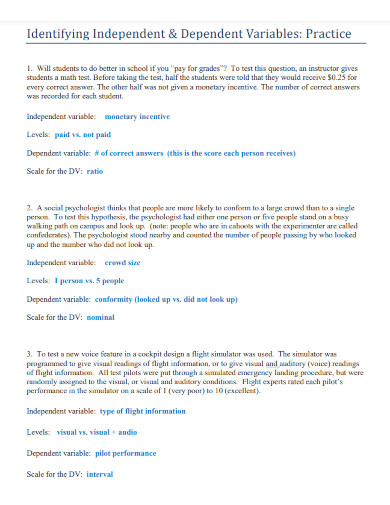jadoon956.files.wordpress.com

## 6. Independent and Dependent Variable Analysis Draft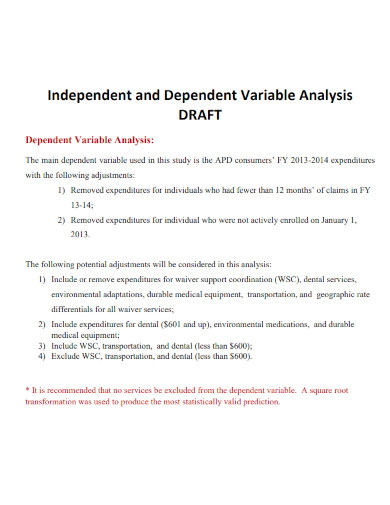apd.myflorida.com

## 7. Proportions as Dependent Variable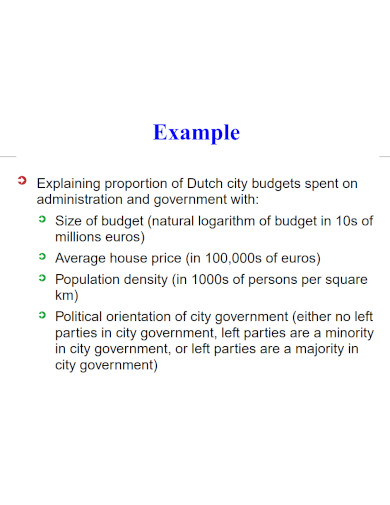repec.org

## 8. Independent and Dependent Variable Examples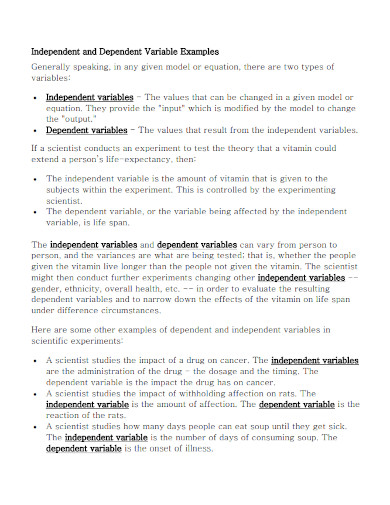images.pcmac.org

## 9. Operationalising Dependent Variable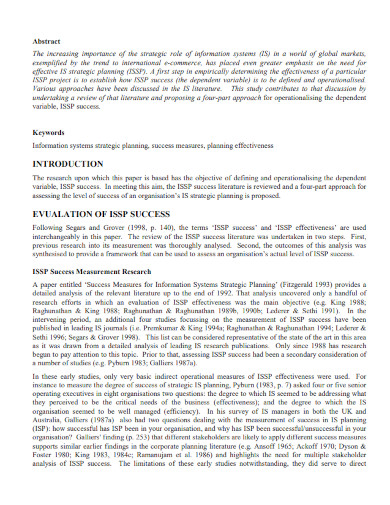aisel.aisnet.org

## 10. Experimental Dependent VariableDesign Templateocsef.org

## 11. Dependent Variable Logistic Regressionstatisticssolutions.com

## 12. Dependent Variable Presentation of Regression Resultscsus.edu

## What Is a Dependent Variable

A dependent variable is a type of variable that refers to a specific element in a research statement. The relationship between the independent and dependent variables is the focal point of the research. The dependent variable will determine what research method you will use during the research proper. If the dependent variable creates an inductive topic, then the person will use qualitative research methods. If the topic requires the researcher to use deduction then they will use quantitative research methods. (see)

## How to Differentiate the Dependent from the Independent Variable

Every good research needs to have a dependent variable that is grounded as it will provide one of the basic structures in the research. This means that if a person wants to study various theses and published journal articles then they must know how to identify the dependent variable from the independent.

### Step 1: Identify the Variables

Begin by identifying the given variables in the question or problem. This will help you easily visualize the differences between both types of variables.

### Step 2: Differentiate the Given Variables

After you have identified all the given variables in the research question or problem, then you must differentiate and isolate each of the variables. Separating these variables will help you differentiate between each variable and determine the specific type of all the given variables. You can also break these variables into specific parts of an outline.

### Step 3: Predict Whether One of the Variables Affects the Other Variable

When you have isolated the variables, you must now check whether or not the modification of one of the variables will affect the other. If the variable affects another variable, it is considered an independent variable, but if the variable does not affect the other variable and is affected, it is a dependent variable.

### Step 4: Repeat Step 3 for All Variables

If there are still variables left in the problem that has not yet been identified, then you must repeat step 3 until you have finished investigating the remaining variables. Do note that there are cases where there is more than one independent and dependent variable.

## Independent variable vs. dependent variables; what is the difference between a dependent and independent variables?

A dependent variable is a variable in a specific experiment, research, or study that is entirely affected by a factor in the experiment called an independent variable. The independent variable is a variable that is not affected by any variables inside the experiment, which is the complete juxtaposition of the dependent variable. The independent variable is the variable that the researchers believe to have a correlational relationship with the dependent variable.

## Why are independent and dependent variables important?

For the modern iteration of the scientific process to occur, at least a single independent and dependent variable must be present in the research article. These variables explain the overall research topic and will dictate the angle and the trajectory of the methodology present in the research effort.

## How many dependent variables do you want in an experiment?

The independent variable determines how the dependent variable changes, thus the name. When performing experiments, researchers aim to quantify the link between the dependent and independent variables. An experiment can have two or more dependent variables, but this is very dependent on the overall topic of the research.

A dependent variable is an element in a research paper or topic affected by the independent variable. Identifying and creating the perfect dependent variable/s will ensure that the research topic has solid ground. The existence of a dependent variable is important in the field of psychology, as dependent variables will determine whether the experiment or research will be a quantitative or a qualitative research topic.